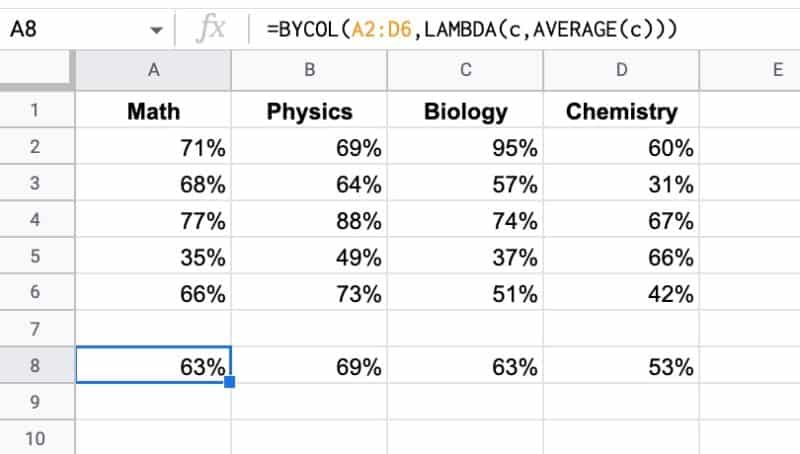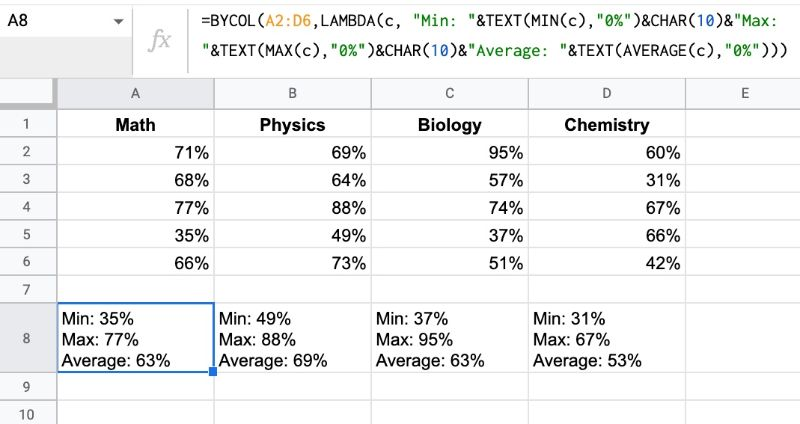# BYCOL Function in Google Sheets – LAMBDA Helper Function

The BYCOL function in Google Sheets operates on an array or range and returns a new row array, created by grouping each column to a single value.

Here’s a simple example, showing a table of exam scores:The formula in A8 is:

`=BYCOL(A2:D6,LAMBDA(c,AVERAGE(c)))`

The easiest way to think about using BYCOL is to think “what function can I use on a single column?” and then think of BYCOL as just repeating that operation across multiple columns.

BYCOL passes the input array to a lambda function, which takes an input column, called “c”, and calculates the average value for that column. It loops over each column and returns a row array of average values, one for each column.

🔗 Get this example and others in the template at the bottom of this article.

## BYCOL Function Syntax

`=BYCOL(array_or_range, lambda)`

It takes two arguments:

`array_or_range`

This is the input array or range you want to group by columns.

`lambda`

This is the custom lambda function that is applied to each column. It must have exactly one name argument and one function expression. The name argument resolves to the current column.

### BYCOL Function Notes

If you give the BYCOL function an input array with M rows and N columns, the output array will be a single row array with N columns.

## BYCOL Formula Example

Let’s extend that first example and work with a more complex lambda expression.

Using the same dataset, we can calculate the minimum, maximum, and average exam scores for all the columns with a single BYCOL function:

`=BYCOL(A2:D6,LAMBDA(c, "Min: "&TEXT(MIN(c),"0%")&CHAR(10)&"Max: "&TEXT(MAX(c),"0%")&CHAR(10)&"Average: "&TEXT(AVERAGE(c),"0%")))`

Again, “c” represents each column in the array.

We calculate each metric and use a CHAR function, CHAR(10), to insert a line break into the string output.

The result is a row showing the min, max, and average for each column:## BYCOL Function Template

Feel free to make a copy: File > Make a copy…

If you can’t access the template, it might be because of your organization’s Google Workspace settings.

In this case, right-click the link to open it in an Incognito window to view it.

1.Ben Ponderin says: